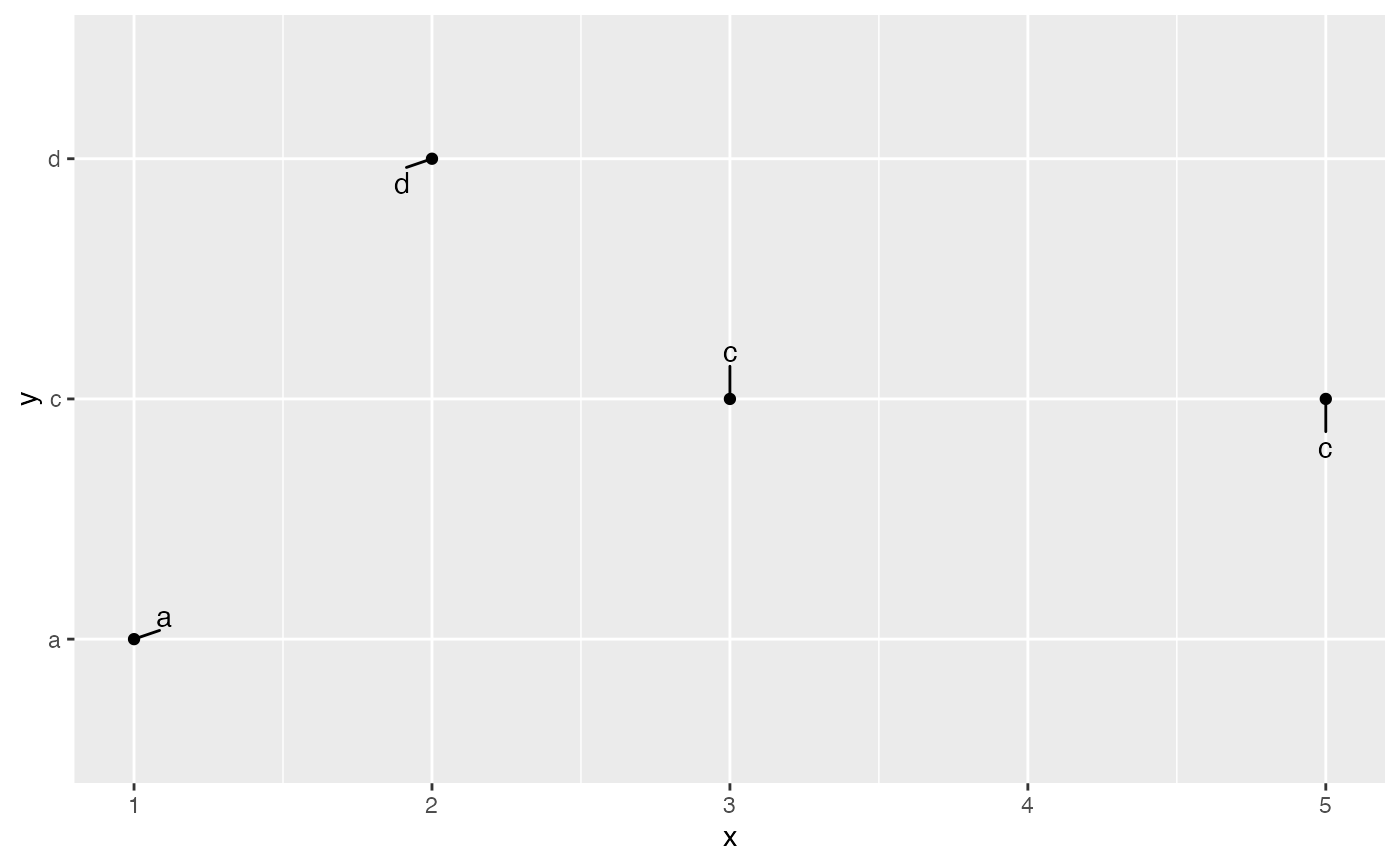position_nudge_repel is useful for adjusting the starting position of text labels before they are repelled from data points.

## Usage

position_nudge_repel(x = 0, y = 0)

## Arguments

x, y

Amount of horizontal and vertical distance to move. Same units as the data on the x and y axes.

## Examples


df <- data.frame(
x = c(1,3,2,5),
y = c("a","c","d","c")
)

ggplot(df, aes(x, y)) +
geom_point() +
geom_text_repel(aes(label = y))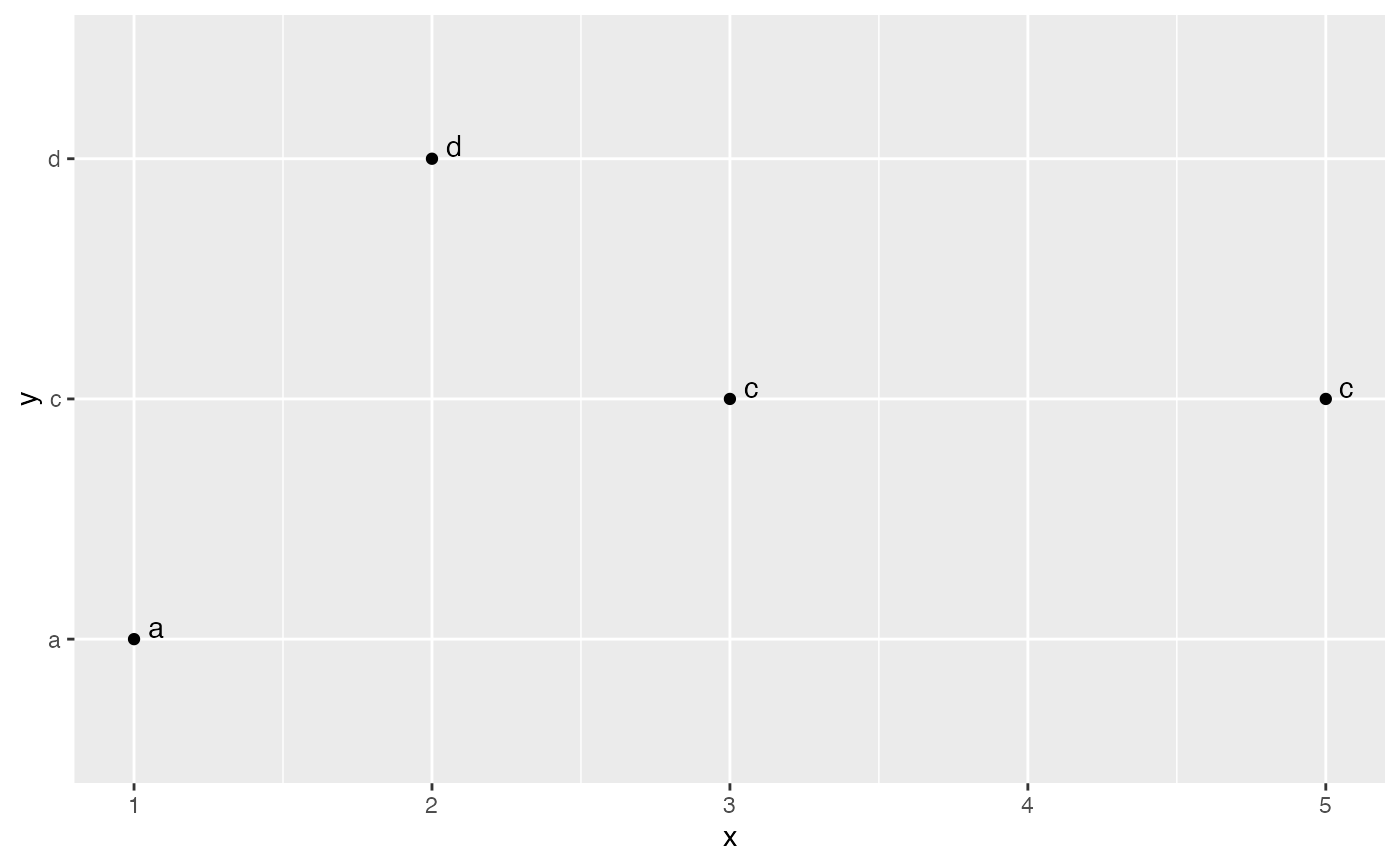ggplot(df, aes(x, y)) +
geom_point() +
geom_text_repel(
aes(label = y),
min.segment.length = 0,
position = position_nudge_repel(x = 0.1, y = 0.15)
)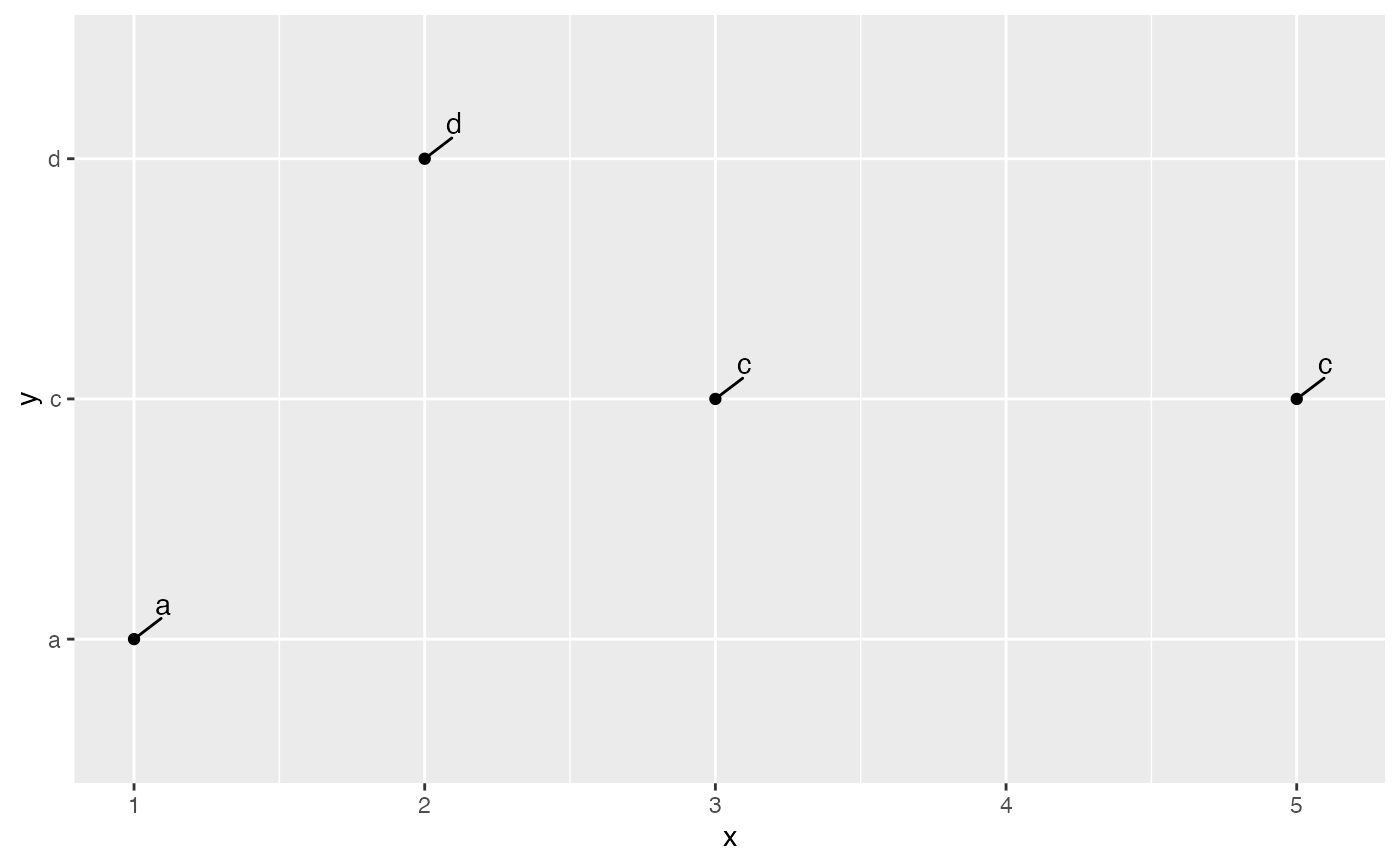# The values for x and y can be vectors
ggplot(df, aes(x, y)) +
geom_point() +
geom_text_repel(
aes(label = y),
min.segment.length = 0,
position = position_nudge_repel(
x = c(0.1, 0, -0.1, 0),
y = c(0.1, 0.2, -0.1, -0.2)
)
)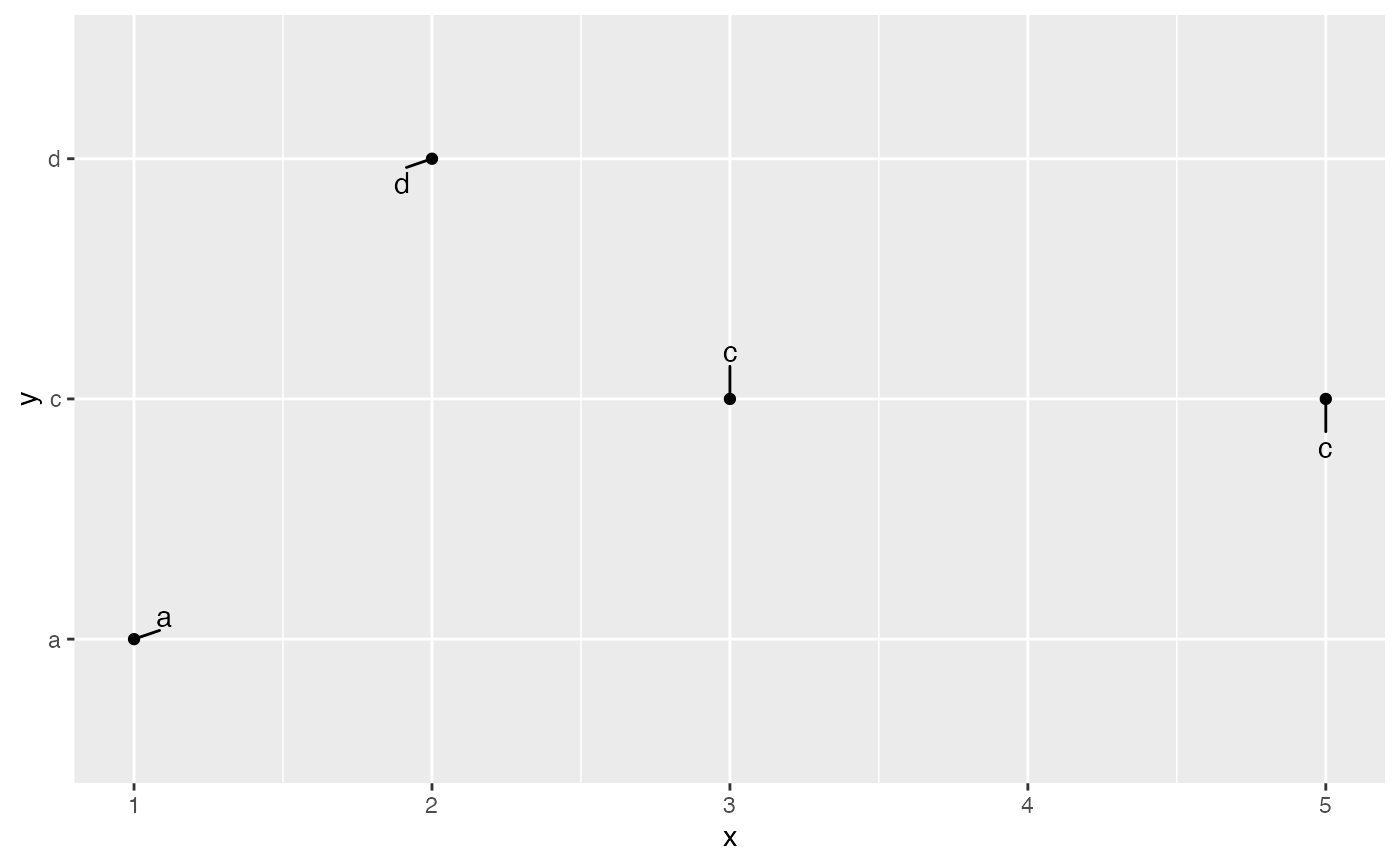# We can also use geom_text_repel() with arguments nudge_x, nudge_y
ggplot(df, aes(x, y)) +
geom_point() +
geom_text_repel(
aes(label = y),
min.segment.length = 0,
nudge_x = 0.1,
nudge_y = 0.15
)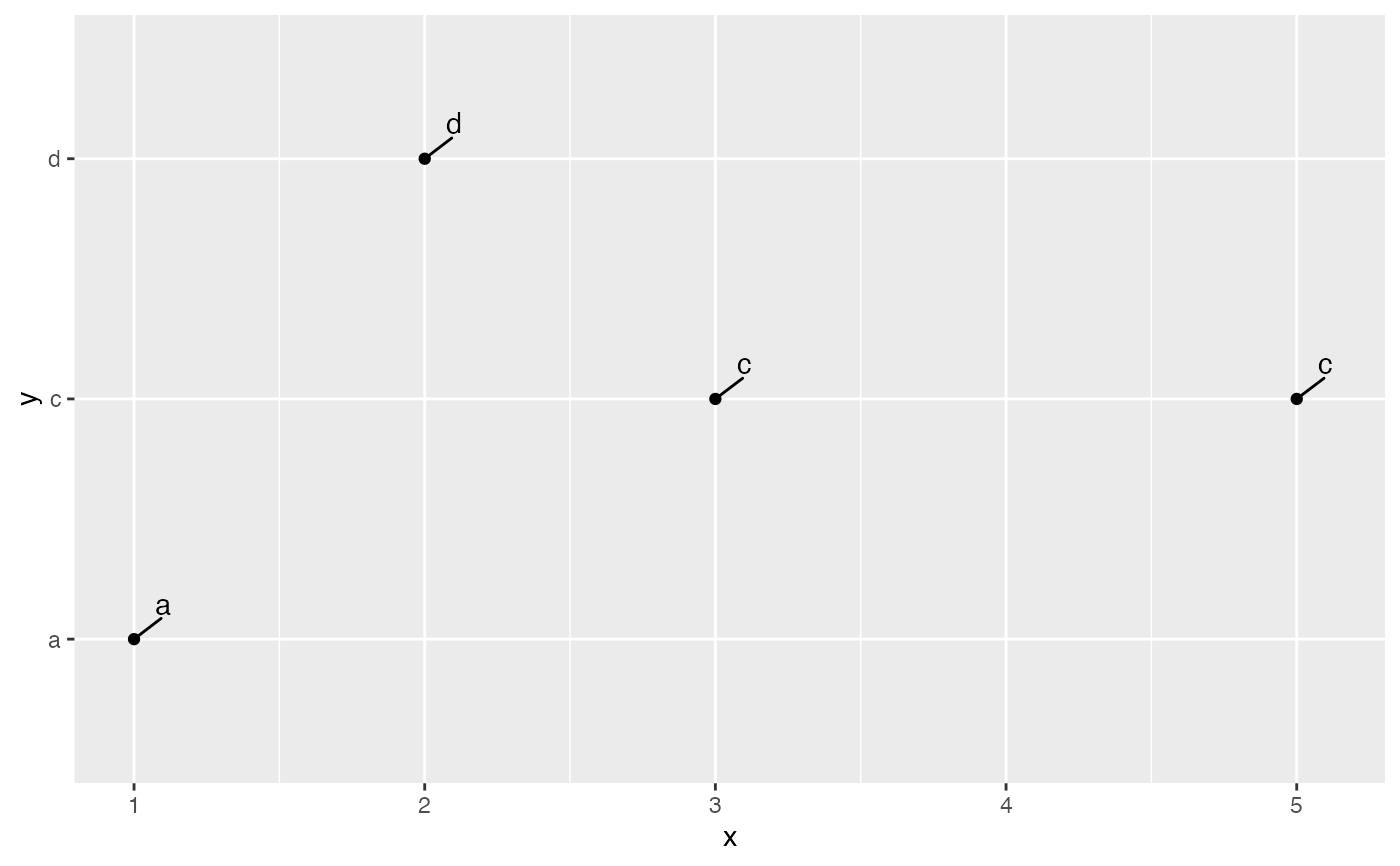# The arguments nudge_x, nudge_y also accept vectors
ggplot(df, aes(x, y)) +
geom_point() +
geom_text_repel(
aes(label = y),
min.segment.length = 0,
nudge_x = c(0.1, 0, -0.1, 0),
nudge_y = c(0.1, 0.2, -0.1, -0.2)
)